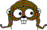Interesting Functions

Hardest n-input for (AND, OR, XOR)
(1) 1
(2) x+y = 1
(3) x+y+z = 1
(4) x+y+z+w = 1
(5) x+y+z+w+v in 0,3

Hardest n-input for (AND, OR)
(1) 1
(2) x^y
(3) x^y^z
(4) x^y^z^w
(5) x+y+z+w+v in 0,1,3,
x+y+z+w+v in 0,1,3 || x&y&z&w&!v,
x+y+z+w+v in 1,3 || x&y&z&w&!v

# Boolean Oracle

This web server computes a minimal Boolean formula for a given function, using the operator sets (AND, OR, XOR) and (AND, OR).

Query: x^y^z (truth table 0xf00f0ff0, canonical 0x0ff0f00f)

A minimal Boolean formula using AND, OR, and XOR requires 2 operators. One such formula is:

¬x ^ ¬y ^ z

A minimal Boolean formula using AND and OR requires 9 operators. One such formula is:

(z & ((x & y) | (¬x & ¬y))) | (¬z & (¬x | ¬y) & (x | y))

How does this work?